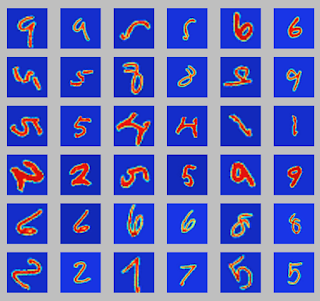### Train a Convolutional Neural Network for Regression

Hello,

I spent the last period working mostly on Tensorflow, studying the APIs and writing some examples in order to explore the possible implementations of neural networks. For this goal, I chose an interesting example proposed in the Matlab examples at . The dataset is composed by 5000 images, rotated by an angle α, and a corresponding integer label (the rotation angle α). The goal is to make a regression to predict the angle of a rotated image and straighten it up.
All files can be found in tests/examples/cnn_linear_model in my repo .

I have kept the structure as in the Matlab example, but I generated a new dataset starting from LeCun's MNIST digits (datasets at ). Each image was rotated by a random angle between 0° and 70°, in order to keep the right orientation of the digits (code in dataset_generation.m). In Fig. 1 some rotated digits with the corresponding original digits.Figure 1. rotated images in columns 1,3,5 and originals in columns 2,4,6

The implemented linear model is:
$\hat{Y} = \omega X + b$,
where the weights $\omega$ and the bias $b$ will be optimized during the training minimizing a loss function. As loss function, I used the mean square error (MSE):
$\dfrac{1}{n} \sum_{i=1}^n (\hat{Y_i} - Y_i)^2$,
where the $Y_i$ are the training labels.

In order to show the effective improvement given by a Neural Network, I started to make a simple regression feeding the X variable of the model directly with the 28x28 images. Even if for the MSE minimization a close form exists, I implemented an iterative method for discovering some Tensorflow features (code in regression.py). For evaluate the accuracy of the regression, I consider a correct regression if the difference between angles is less than 20°. After 20 epochs, the convergence was almost reached, giving an accuracy of $0.6146$.Figure 2. rotated images in columns 1,3,5 and after the regression in columns 2,4,6

I want to analyze now the improvement given by a feature extraction performed with a convolutional neural network (CNN). As in the Matlab example, I used a basic CNN since the input images are quite simple (only numbers with monochromatic background) and consequently the features to extract are few.
• INPUT [28x28x1] will hold the raw pixel values of the image, in this case an image of width 28, height 28
• CONV layer will compute the output of neurons that are connected to local regions in the input, each computing a dot product between their weights and a small region they are connected to in the input volume. This results in volume such as [12x12x25]: 25 filters of size 12x12
• RELU layer will apply an element-wise activation function, such as the $max(0,x)$ thresholding at zero. This leaves the size of the volume unchanged ([12x12x25]).
• FC (i.e. fully-connected) layer will compute the class scores, resulting in volume of size [1x1x1], which corresponds to the rotation angle. As with ordinary Neural Networks, each neuron in this layer will be connected to all the numbers in the previous volume.Figure 3. CNN linear model architecture

We can visualize the architecture with Tensorboard where the graph of the model is represented.Figure 4. Model graph generated with Tensorboard

With the implementation in regression_w_CNN.py, the results are quite satisfying: after 15 epochs, it reached an accuracy of $0.75$ (205 seconds overall). One can see in Fig. 4 the marked improvement of the regression.Figure 5. rotated images in columns 1,3,5 and after the CNN regression in columns 2,4,6
With the same parameters, Matlab reached an accuracy of $0.76$ in 370 seconds (code in regression_Matlab_nnet.m), so the performances are quite promising

In the next post (in few days), I will integrate the work done up to now, calling the Python class within Octave and making a function that simulates the behavior of Matlab. Leveraging the layers classes that I made 2 weeks ago, I will implement a draft of the functions trainNetwork and predict making the Matlab script callable also in Octave.

I will also care about the dependencies of the package: I will add the dependency from Pytave in the package description and write a test as PKG_ADD in order to verify the version of Tensorflow during the installation of the package.

Peace,
Enrico
 http://yann.lecun.com/exdb/mnist/